# Math

• Distances
• Trigonometry
• Interpolation

# Pythagorean Theorem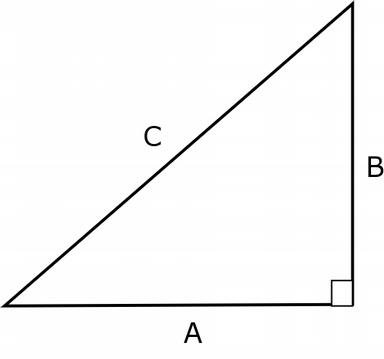A*A + B*B = C*C

# Cartesian Coordinates# Euclidean Distance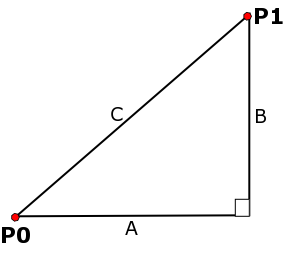Distance between two points P0 (x0,y0) and P1 (x1,y1):

```   A = x1 - x0
B = y1 - y0

(x1-x0)*(x1-x0) + (y1-y0)*(y1-y0) = C*C

dist = C = sqrt((x1-x0)*(x1-x0) + (y1-y0)*(y1-y0))
```

Distance between two 3D points P0 (x0,y0,z0) and P1 (x1,y1,z1):

```   dist = sqrt((x1-x0)*(x1-x0) + (y1-y0)*(y1-y0) + (z1-z0)*(z1-z0))
```

# Comparing Distances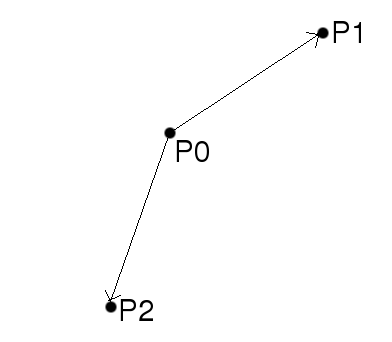sqrt is considered an expensive (i.e. slow) function

Avoid using it if you can
For example, to determine which of 2 points (P1 or P2) is closer to point P0:

```  dist1 = (x1-x0)*(x1-x0) + (y1-y0)*(y1-y0) + (z1-z0)*(z1-z0)
dist2 = (x2-x0)*(x2-x0) + (y2-y0)*(y2-y0) + (z2-z0)*(z2-z0)
if dist1 < dist2:
P1 is closer
else:
P2 is closer
```

# Trigonometry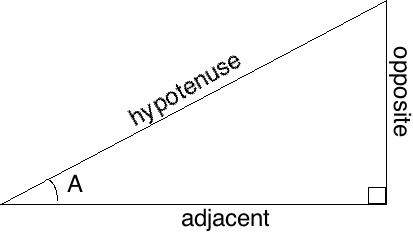```sin(A) = opposite / hypotenuse
```

or

```opposite = hypotenuse * sin(A)
```

# Trigonometry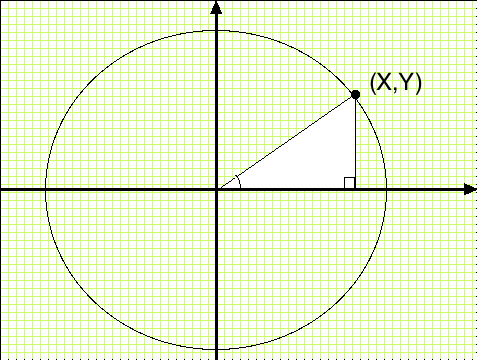```x = radius * cos(A)
```

Standard math library functions use radians

360 degrees = 1 full circle = 2 π radians

(Circumference of unit circle = 2 π)

```    radians = degrees / 360.0 * 2 * pi
```

or

```    radians = degrees / 180.0 * pi
```
(or use Python's pre-defined functions math.radians(d) and math.degrees(r))

# Making a Circle

```    vertices = []
for degrees in range(0, 360):
vertices += [x,y]
vlist = pyglet.graphics.vertex_list(360, ('v2f', vertices))
```

# Driving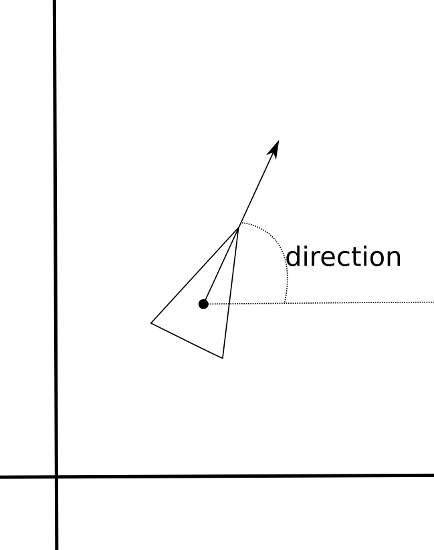Vehicle has direction and speed of travel

Direction is orientation - rotation about Z

To move forward:

```    distance = speed * time

dx = math.cos(direction) * distance
dy = math.sin(direction) * distance

x = x + dx
y = y + dy
```

# atan2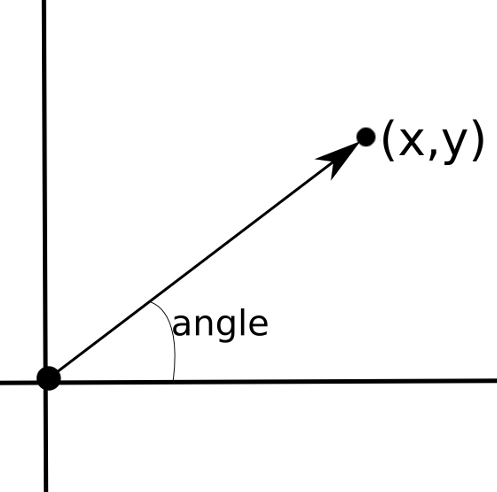atan2 converts from (X,Y) coordinates back to angles

Note: it takes arguments in the order Y, X

```
angle = math.degrees( math.atan2(y,x) )
```

# Interpolation

Deriving a value for something from two pre-defined values (extremes)

e.g. Moving object from one position to another, over time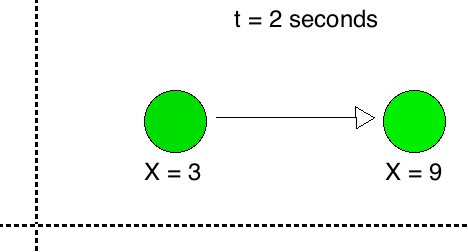# Linear Interpolation

Interpolation expressed as fractional distance between the two extremes

Ranges from 0.0 to 1.0
0.0 = first point; 1.0 = second point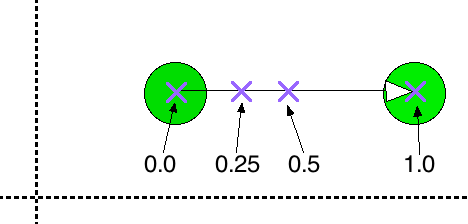# Linear Interpolation

For a single value, with extremes V0 & V1 and interpolation fraction A:

```    V = (1 - A) * V0 + A * V1
```

For multiple values, such as XYZ position, use the same fraction A for all:

```    X = (1 - A) * X0 + A * X1
Y = (1 - A) * Y0 + A * Y1
Z = (1 - A) * Z0 + A * Z1
```

# Timing

To interpolate over time, compute interpolation fraction based on the amount of time that has passed.

Example:

```    def startAnimation():
animating = True
startTime = time.time()
duration = 5

def computeAnimation():
if animating:
t = time.time() - startTime
if t <= duration:
a = t / duration
else:
animating = False
a = 1
x = (1-a)*startX + a*endX
y = (1-a)*startY + a*endY
z = (1-a)*startZ + a*endZ
```

# Timing

Linear Slow-in Slow-out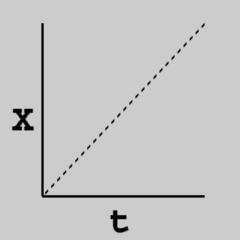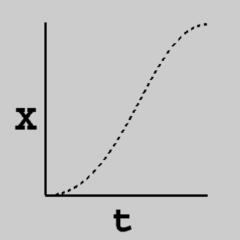X = tX = -2*t*t*t + 3*t*t
```A2 = -2*A*A*A + 3*A*A
V = (1-A2) * V0 + A2 * V1
```This document is by Dave Pape, and is released under a Creative Commons License.